# Freight and passenger car

The truck starts at 8 pm at 30 km/h. Passenger car starts at 8 pm at 40 km/h. Passenger arrives in the destination city 1 hour and 45 min earlier. What is the distance between the city of departure and destination city?

Result

s =  210 km

#### Solution:

30 t = 40 (t-1.75)
s = 30 t

10t = 70
s-30t = 0

s = 210
t = 7

Calculated by our linear equations calculator.

Leave us a comment of example and its solution (i.e. if it is still somewhat unclear...):Be the first to comment!#### To solve this example are needed these knowledge from mathematics:

Do you want to convert length units?

## Next similar examples:

1. Truck and car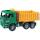At 6h45min the truck started at a speed of 36 km/h. At 7h30m behind it a passenger car. Find the average speed of passenger car if it will catch truck 72 km away from the factory.
2. FailureDrivers of passenger cars have calculated that at a speed of 60 km/h arrives at destination within 40 minutes. After 20 km refrain five minutes due a technical failure. How fast must go the rest of the way to the finish came at the scheduled time?
3. Two citiesCities A and B are 200 km away. At 7 o'clock from city A, the car started at an average speed of 80 km/h, and from B at 45 min later the motorcycle is started at an average speed of 120 km/h. How long will they meet and at what distance from the point A it
4. Cars 2Opava is 360 km distance from Prešov. At 8:00 hour two cars started against each other from this cities at average speeds 88 km/h and 121 km/h. At what hour its will meet and what distance its travel if car started from Prešov is faster?
5. Cars 6At 9:00 am two cars started from the same town and traveled at a rate of 35 miles per hour and the other car traveled at a rate of 40 miles per hour. After how many hours will the cars be 30 miles apart?
6. Steamer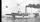At 6 hours 40 minutes steamer sailed from the port at speed 12 km/h. At exactly 10 hours started sail motorboat at speed 42 km/h. When motorboat will catch steamer?
7. Pedestrian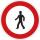Pedestrian came started at 8h in the morning with speed 4.4 km/h. At half-past eleven cyclist started at 26 km/h same way. How many minutes take cyclist to catch up pedestrian?
8. Round-tripA woman works at a law firm in city A which is about 50 miles from city B. She must go to the law library in city B to get a document. Find how long it takes her to drive​ round-trip if she averages 40 mph.
9. Bob traveled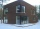Bob traveled 20 meters to Dave's house. He arrived in 3 minutes. What was his average speed for the trip.
10. StormSo far, a storm has traveled 35 miles in 1/2 hour in direction straight to observer. If it is currently 5:00 p. m. and the storm is 105 miles away from you, at what time will the storm reach you? Explain how you solved the problem.
11. Moving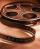Vojta left the house at three o'clockat 4 km/h. After half hour later went from the same place Filip by bicycle at speed 18 km/h. How long take Tilip to catch up Vojta and how far from the house?
12. Scale of mapJames travels one kilometer in 12 minutes. The route walked for half an hour measured on the map 5 cm. Calculate how many kilometers James walked for half an hour. Find the scale of the map.
13. Skier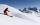At this point, the first skier lead 20 km before the second skier and travels at a constant speed 19 km/h. The second skier rides at 24 km/h. How long take him to catch up the first?
14. Wave parameters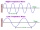Calculate the speed of a wave if the frequency is 336 Hz and the wavelength is 10 m.
15. WalkersWalker, which makes 120 steps per minute, make distance from point A to point B for 55 minutes. The length of his step is 75cm. For how long does this distance go boy who will do 110 steps 60 cm long in a minute?
16. Days2minutesWrite a formula that converts x days to y minutes. The formula is:
17. Aircraft nose downHow long will fall airliner from a height of 10000 m at speed 1,000 km/h?